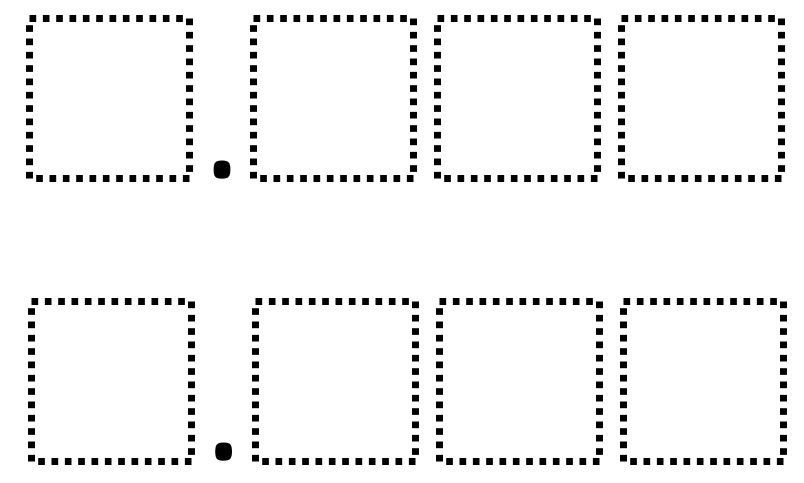# Rounding Decimals 3

Directions: Using the digits 0 to 9 at most one time each, place a digit in each box to create two different decimals that are equivalent when rounded to the nearest tenth and have the least possible value.### Hint

How can you have values that are equivalent when rounded to the nearest tenth yet have different whole number values?

There are many possible answers which all round to 2.0 including 1.978 and 2.043.

Source: Robert Kaplinsky

## Add Fractions with Decimal Sums

Directions: Using the digits 1 to 9 at most one time each, place a digit …

1.Shouldn’t the directions say “round to the nearest whole number” if the example you gave rounds both to 2?

•I was wondering this. If I can use 0, why can’t I round to 1? 0.637 and 1.524

2.1.968 and 2.075 (5th grade class in NJ)

•If the decimal numbers have to have the least possible value, should there not just be one ansswer instead of multiple ones?

Would 1.956 and 2.034 be the least possible values that round to 2.0?

3.Just kidding…. that should say 1.968 and 2.035

4.Sarah Smith and John Smith

we really liked the challenge

5.1.986 and 2.034 both round to 2.0

6.yes…but when rounded to the nearest tenth they both are 2.0. Good discussion point!

7.0.356
1.427

8.We found that 1.956 and 2.043 both round to 2.0 🙂